# Tutorial

#### 2020-11-09

The package morse is devoted to the analysis of data from standard toxicity tests. It provides a simple workflow to explore/visualize a data set, and compute estimations of risk assessment indicators. This document illustrates a typical use of morse on survival and reproduction data, which can be followed step-by-step to analyze new data sets.

# Survival data analysis at target time (TT)

The following example shows all the steps to perform survival analysis on standard toxicity test data and to produce estimated values of the $$LC_x$$. We will use a data set of the library named cadmium2, which contains both survival and reproduction data from a chronic laboratory toxicity test. In this experiment, snails were exposed to six concentrations of a metal contaminant (cadmium) during 56 days.

## Step 1: check the structure and the data set integrity

The data from a survival toxicity test should be gathered in a data.frame with a specific layout. This is documented in the paragraph on survData in the reference manual, and you can also inspect one of the data sets provided in the package (e.g., cadmium2). First, we load the data set and use the function survDataCheck() to check that it has the expected layout:

data(cadmium2)
survDataCheck(cadmium2)
## No message

The output ## No message just informs that the data set is well-formed.

## Step 2: create a survData object

The class survData corresponds to survival data and is the basic layout used for the subsequent operations. Note that if the call to survDataCheck() reports no error (i.e., ## No message), it is guaranteed that survData will not fail.

dat <- survData(cadmium2)
head(dat)
## # A tibble: 6 x 6
##    conc  time Nsurv Nrepro replicate Ninit
##   <dbl> <int> <int>  <int>     <dbl> <int>
## 1     0     0     5      0         1     5
## 2     0     3     5    262         1     5
## 3     0     7     5    343         1     5
## 4     0    10     5    459         1     5
## 5     0    14     5    328         1     5
## 6     0    17     5    742         1     5

## Step 3: visualize your data set

The function plot() can be used to plot the number of surviving individuals as a function of time for all concentrations and replicates.

plot(dat, pool.replicate = FALSE)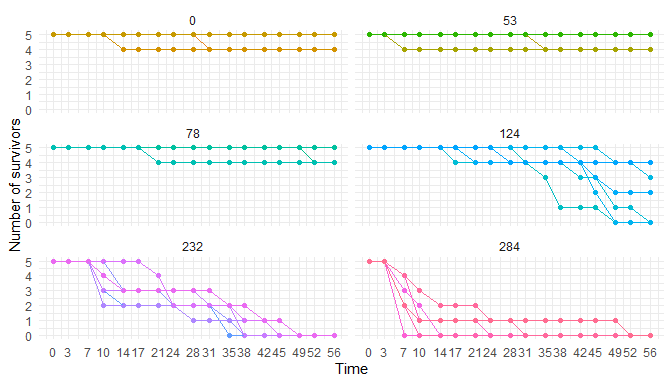Two graphical styles are available, "generic" for standard R plots or "ggplot" to call package ggplot2 (default). If argument pool.replicate is TRUE, datapoints at a given time-point and a given concentration are pooled and only the mean number of survivors is plotted. To observe the full data set, we set this option to FALSE.

By fixing the concentration at a (tested) value, we can visualize one subplot in particular:

plot(dat, concentration = 124, addlegend = TRUE,
pool.replicate = FALSE, style ="generic")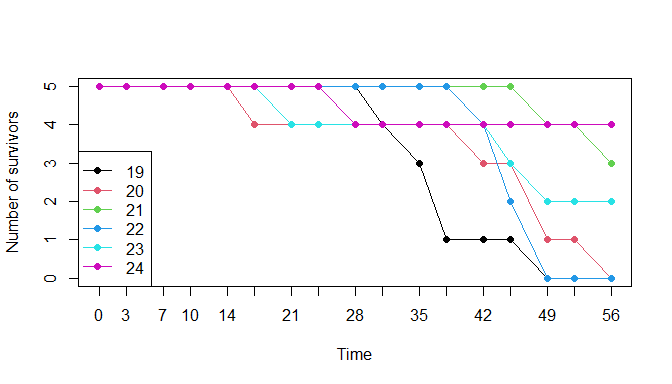We can also plot the survival rate, at a given time-point, as a function of concentration, with binomial confidence intervals around the data. This is achieved by using function plotDoseResponse() and by fixing the option target.time (default is the end of the experiment).

plotDoseResponse(dat, target.time = 21, addlegend = TRUE)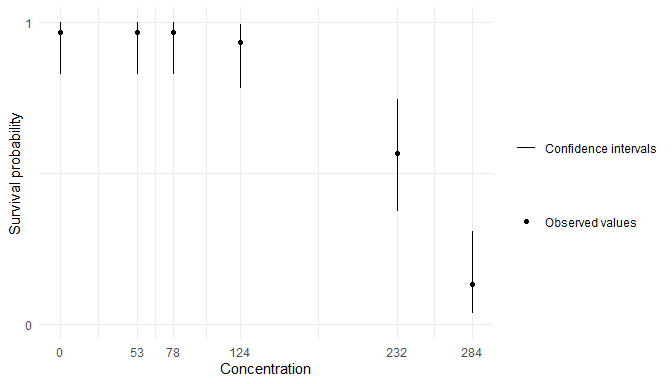Function summary() provides some descriptive statistics on the experimental design.

summary(dat)
##
## Number of replicates per time and concentration:
##      time
## conc  0 3 7 10 14 17 21 24 28 31 35 38 42 45 49 52 56
##   0   6 6 6  6  6  6  6  6  6  6  6  6  6  6  6  6  6
##   53  6 6 6  6  6  6  6  6  6  6  6  6  6  6  6  6  6
##   78  6 6 6  6  6  6  6  6  6  6  6  6  6  6  6  6  6
##   124 6 6 6  6  6  6  6  6  6  6  6  6  6  6  6  6  6
##   232 6 6 6  6  6  6  6  6  6  6  6  6  6  6  6  6  6
##   284 6 6 6  6  6  6  6  6  6  6  6  6  6  6  6  6  6
##
## Number of survivors (sum of replicates) per time and concentration:
##      0  3  7 10 14 17 21 24 28 31 35 38 42 45 49 52 56
## 0   30 30 30 30 29 29 29 29 29 28 28 28 28 28 28 28 28
## 53  30 30 29 29 29 29 29 29 29 29 28 28 28 28 28 28 28
## 78  30 30 30 30 30 30 29 29 29 29 29 29 29 29 29 27 27
## 124 30 30 30 30 30 29 28 28 27 26 25 23 21 18 11 11  9
## 232 30 30 30 22 18 18 17 14 13 12  8  4  3  1  0  0  0
## 284 30 30 15  7  4  4  4  2  2  1  1  1  1  1  1  0  0

## Step 4: fit an exposure-response model to the survival data at target time

Now we are ready to fit a probabilistic model to the survival data, in order to describe the relationship between the concentration in chemical compound and survival rate at the target time. Our model assumes this latter is a log-logistic function of the former, from which the package delivers estimates of the parameters. Once we have estimated the parameters, we can then calculate the $$LC_x$$ values for any $$x$$. All this work is performed by the survFitTT() function, which requires a survData object as input and the levels of $$LC_x$$ we want:

fit <- survFitTT(dat,
target.time = 21,
lcx = c(10, 20, 30, 40, 50))

The returned value is an object of class survFitTT providing the estimated parameters as a posterior1 distribution, which quantifies the uncertainty on their true value. For the parameters of the models, as well as for the $$LC_x$$ values, we report the median (as the point estimated value) and the 2.5 % and 97.5 % quantiles of the posterior (as a measure of uncertainty, a.k.a. credible intervals). They can be obtained by using the summary() method:

summary(fit)
## Summary:
##
## The  loglogisticbinom_3  model with a binomial stochastic part was used !
##
## Priors on parameters (quantiles):
##
##         50%      2.5%     97.5%
## b 1.000e+00 1.259e-02 7.943e+01
## d 5.000e-01 2.500e-02 9.750e-01
## e 1.227e+02 5.390e+01 2.793e+02
##
## Posteriors of the parameters (quantiles):
##
##         50%      2.5%     97.5%
## b 8.572e+00 4.058e+00 1.596e+01
## d 9.569e-01 9.089e-01 9.862e-01
## e 2.364e+02 2.097e+02 2.538e+02
##
## Posteriors of the LCx (quantiles):
##
##            50%      2.5%     97.5%
## LC10 1.831e+02 1.270e+02 2.139e+02
## LC20 2.013e+02 1.541e+02 2.261e+02
## LC30 2.143e+02 1.747e+02 2.353e+02
## LC40 2.256e+02 1.927e+02 2.439e+02
## LC50 2.364e+02 2.097e+02 2.538e+02

If the inference went well, it is expected that the difference between quantiles in the posterior will be reduced compared to the prior, meaning that the data were helpful to reduce the uncertainty on the true value of the parameters. This simple check can be performed using the summary function.

The fit can also be plotted:

plot(fit, log.scale = TRUE, adddata = TRUE,   addlegend = TRUE)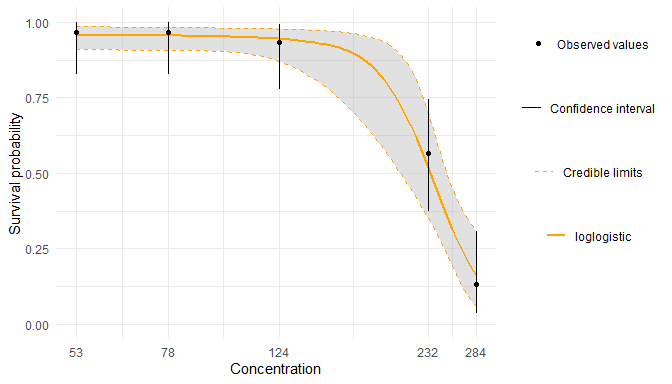This representation shows the estimated relationship between concentration of chemical compound and survival rate (orange curve). It is computed by choosing for each parameter the median value of its posterior. To assess the uncertainty on this estimation, we compute many such curves by sampling the parameters in the posterior distribution. This gives rise to the grey band, showing for any given concentration an interval (called credible interval) containing the survival rate 95% of the time in the posterior distribution. The experimental data points are represented in black and correspond to the observed survival rate when pooling all replicates. The black error bars correspond to a 95% confidence interval, which is another, more straightforward way to bound the most probable value of the survival rate for a tested concentration. In favorable situations, we expect that the credible interval around the estimated curve and the confidence interval around the experimental data largely overlap.

A similar plot is obtained with the style "generic":

plot(fit, log.scale = TRUE, style = "generic", adddata = TRUE, addlegend = TRUE)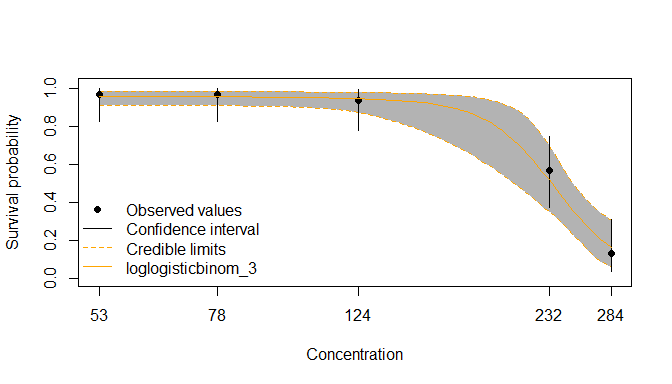Note that survFitTT() will warn you if the estimated $$LC_{x}$$ lie outside the range of tested concentrations, as in the following example:

data("cadmium1")
target.time = 21,
lcx = c(10, 20, 30, 40, 50))
## Warning: The LC50 estimation (model parameter e) lies outside the range of
## tested concentrations and may be unreliable as the prior distribution on this
## parameter is defined from this range !
plot(doubtful_fit, log.scale = TRUE, style = "ggplot", adddata = TRUE,
addlegend = TRUE)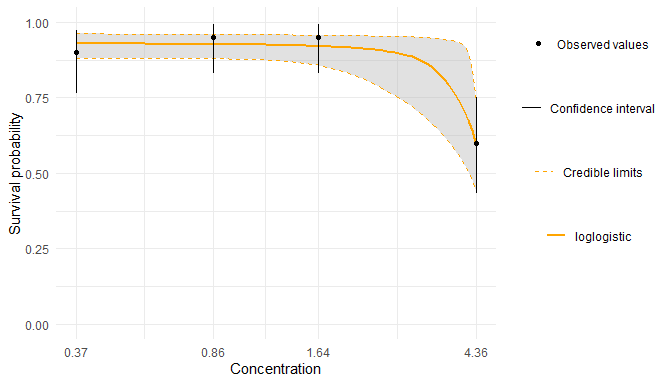In this example, the experimental design does not include sufficiently high concentrations, and we are missing measurements that would have a major influence on the final estimation. For this reason this result should be considered unreliable.

## Step 5: validate the model with a posterior predictive check

The fit can be further validated using so-called posterior predictive checks: the idea is to plot the observed values against the corresponding estimated predictions, along with their 95% credible interval. If the fit is correct, we expect to see 95% of the data inside the intervals.

ppc(fit)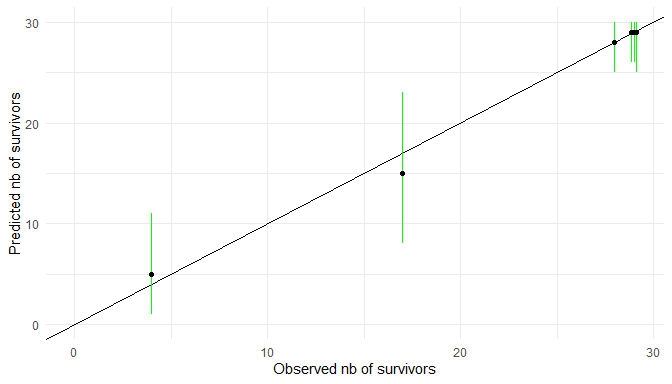In this plot, each black dot represents an observation made at a given concentration, and the corresponding number of survivors at target time is given by the value on the x-axis. Using the concentration and the fitted model, we can produce the corresponding prediction of the expected number of survivors at that concentration. This prediction is given by the y-axis. Ideally observations and predictions should coincide, so we’d expect to see the black dots on the points of coordinate $$Y = X$$. Our model provides a tolerable variation around the predited mean value as an interval where we expect 95% of the dots to be in average. The intervals are represented in green if they overlap with the line $$Y=X$$, and in red otherwise.

# Survival analysis using a toxicokinetic-toxicodynamic (TKTD) model: GUTS

The steps for a TKTD data analysis are absolutely analogous to what we described for the analysis at target time. Here the goal is to estimate the relationship between chemical compound concentration, time and survival rate using the GUTS models. GUTS, for General Unified Threshold models of Survival, is a TKTD models generalising most of existing mechanistic models for survival description. For details about GUTS models, see the vignette theory under modelling approaches, and the included references.

## GUTS model with constant exposure concentrations

Here is a typical session to analyse concentration-dependent time-course data using the so-called “Stochastic Death” (SD) model:

# (1) load data set
data(propiconazole)

# (2) check structure and integrity of the data set
survDataCheck(propiconazole)

# (3) create a survData object
dat <- survData(propiconazole)

# (4) represent the number of survivors as a function of time
plot(dat, pool.replicate = FALSE)

# (5) check information on the experimental design
summary(dat)

### GUTS model “SD”

To fit the Stochastic Death model, we have to specify the model_type as "SD":

# (6) fit the TKTD model SD
fit_cstSD <- survFit(dat, quiet = TRUE, model_type = "SD")

Then, the summary() function provides parameters estimates as medians and 95% credible intervals.

# (7) summary of parameters estimates
summary(fit_cstSD)
# OR

### GUTS model “IT”

The Individual Tolerance (IT) model is a variant of TKTD survival analysis. It can also be used with morse as demonstrated hereafter. For the IT model, we have to specify the model_type as "IT":

fit_cstIT <- survFit(dat, quiet = TRUE, model_type = "IT")

We can first get a summary of the estimated parameters:

summary(fit_cstIT)
# OR
fit_cstIT$estim.par And the plot of posteriors vs. priors distributions: plot_prior_post(fit_cstIT) plot(fit_cstIT) ppc(fit_cstIT) ## GUTS model under time-variable exposure concentration Here is a typical session fitting an SD or an IT model for a data set under time-variable exposure scenario. # (1) load data set data("propiconazole_pulse_exposure") # (2) check structure and integrity of the data set survDataCheck(propiconazole_pulse_exposure) # (3) create a survData object dat <- survData(propiconazole_pulse_exposure) # (4) represent the number of survivor as a function of time plot(dat) # (5) check information on the experimental design summary(dat) ### GUTS model “SD” # (6) fit the TKTD model SD fit_varSD <- survFit(dat, quiet = TRUE, model_type = "SD") # (7) summary of the fit object summary(fit_varSD) plot_prior_post(fit_varSD) plot(fit_varSD) ppc(fit_varSD) ### GUTS model “IT” # fit a TKTD model IT fit_varIT <- survFit(dat, quiet = TRUE, model_type = "IT") # (7) summary of the fit object summary(fit_varIT) plot_prior_post(fit_varIT) plot(fit_varIT) ppc(fit_varIT) ## Computing prediction GUTS models can be used to simulate the survival of the organisms under any exposure pattern, using the calibration done with function survFit() from observed data. The function for prediction is called predict() and returns an object of class survFitPredict. # (1) upload or build a data frame with the exposure profile # argument replicate is used to provide several profiles of exposure data_4prediction <- data.frame(time = c(1:10, 1:10), conc = c(c(0,0,40,0,0,0,40,0,0,0), c(21,19,18,23,20,14,25,8,13,5)), replicate = c(rep("pulse", 10), rep("random", 10))) # (2) Use the fit on constant exposure propiconazole with model SD (see previously) predict_PRZ_cstSD_4pred <- predict(object = fit_cstSD, data_predict = data_4prediction) If NA are produce an error message is returned. From an object survFitPredict, results can ben plotted with function plot(): # (3) Plot the predicted survival rate under the new exposure profiles. plot(predict_PRZ_cstSD_4pred) ### Robust ODE solver with deSolve It appears that with some extreme data set, the fast way used to compute predictions return NA data, due to numerical error (e.g. number greater or lower than $$10^{300}$$ or $$10^{-300}$$). When this issue happens, the function predict() returns an error, with the message providing the way to use the robust implementation with ODE solver provided by deSolve. This way is implemented through the use of the function predict_ode(). Robustness goes often with longer time to compute. Time to compute can be long, so we use by default MCMC chain size of 1000 independent iterations. predict_PRZ_cstSD_4pred_ode <- predict_ode(object = fit_cstSD, data_predict = data_4prediction) This new object predict_PRZ_cstSD_4pred_ode is a survFitPredict object and so it has exactly the same properties as an object returned by a predict() function. Note that since predict_ode() can be very long to compute, the mcmc_size is reduced to 1000 MCMC chains by default. See for instance, with the plot: plot(predict_PRZ_cstSD_4pred_ode) ### Removing background mortality in predictions While the model has been estimated using the background mortality parameter hb, it can be interesting to see the prediction without it. This is possible with the argument hb_value. If TRUE, the background mortality is taken into account, and if FALSE, the background mortality is set to $$0$$ in the prediction. # Use the same data set profile to predict without 'hb' predict_PRZ_cstSD_4pred_hbOUT <- predict_ode(object = fit_cstSD, data_predict = data_4prediction, hb_value = FALSE, hb_valueFORCED = 0) # Plot the prediction: plot(predict_PRZ_cstSD_4pred_hbOUT) # Use the same data set profile to predict without 'hb' predict_PRZ_cstSD_4pred_hbFIX2 <- predict_ode(object = fit_cstSD, data_predict = data_4prediction, hb_value = FALSE, hb_valueFORCED = 0.2) # Plot the prediction: plot(predict_PRZ_cstSD_4pred_hbFIX2) ## Validation criteria: EFSA recommendations Following EFSA recommendations, the next functions compute qualitative and quantitative model performance criteria suitable for GUTS, and TKTD modelling in general: the percentage of observations within the 95% credible interval of the Posterior Prediction Check (PPC), the Normalised Root Mean Square Error (NRMSE) and the Survival-Probability Prediction Error (SPPE). PPC The PPC compares the predicted median numbers of survivors associated to their uncertainty limits with the observed numbers of survivors. This can be visualised by plotting the predicted versus the observed values and counting how frequently the confidence/credible limits intersect with the 1:1 prediction line [see previous plot]. Based on experience, PPC resulting in less than 50% of the observations within the uncertainty limits indicate poor model performance. Normalised Root Mean Square Error NRMSE NRMSE criterion is also based on the expectation that predicted and observed survival numbers matches the 1:1 line in a scatter plot. The criterion is based on the classical root-mean-square error (RMSE), used to aggregate the magnitudes of the errors in predictions for various time-points into a single measure of predictive power. In order to provide a criterion expressed as a percentage, it is suggested using a normalised RMSE by the mean of the observations. $NRMSE = \frac{RMSE}{\overline{Y}} = \frac{1}{\overline{Y}} \sqrt{\frac{1}{n} \sum_{i=1}^{n} (Y_{obs,i} - Y_{pred,i})^2} \times 100$ Survival Probability Prediction Error (SPPE) The SPPE indicator is negative (between 0 and -100%) for an underestimation of effects, and positive (between 0 and 100%) for an overestimation of effects. An SPPE value of 0% means an exact prediction of the observed survival probability at the end of the experiment. $SPPE = \left( \frac{Y_{obs, t_{end}}}{Y_{init}} - \frac{Y_{pred, t_{end}}}{Y_{init}} \right) \times 100 = \frac{Y_{obs, t_{end}} - Y_{pred, t_{end}}}{Y_{init}} \times 100$ ### Computing predictions with number of survivors For NRMSE and SPPE, we need to compute the number of survivors. To do so, we use the function predict_Nsurv() where two arguments are required: the first argument is a survFit object, and the other is a data set with four columns (time, conc, replicate and Nsurv). Contrary to the function predict(), here the column Nsurv is necessary. predict_Nsurv_PRZ_SD_cstTOcst <- predict_Nsurv(fit_cstSD, propiconazole) predict_Nsurv_PRZ_SD_varTOcst <- predict_Nsurv(fit_varSD, propiconazole) predict_Nsurv_PRZ_SD_cstTOvar <- predict_Nsurv(fit_cstSD, propiconazole_pulse_exposure) predict_Nsurv_PRZ_SD_varTOvar <- predict_Nsurv(fit_varSD, propiconazole_pulse_exposure) ### Robust implementation with ODE solver predict_Nsurv_ode For the same reason that a predict_ode function as been implemented to compute predict function using the ODE solver of deSolve, a predict_Nsurv_ode function as been implemented as equivalent to predict_Nsurv. The time to compute is subtentially longer than the original function. predict_Nsurv_PRZ_SD_cstTOcst_ode <- predict_Nsurv_ode(fit_cstSD, propiconazole) When both function work well, their results are identical (or highly similar): plot(predict_Nsurv_PRZ_SD_cstTOcst) plot(predict_Nsurv_PRZ_SD_cstTOcst_ode) plot(predict_Nsurv_PRZ_SD_cstTOcst_ode) ### Validation criteria Then, using object produce with the function predict_Nsurv() we can compute PPC, NRMSE and SPPE for all models. predict_Nsurv_check(predict_Nsurv_PRZ_SD_cstTOvar) ### Plot and PPC of predict_Nsurv objects plot(predict_Nsurv_PRZ_SD_cstTOvar) When ploting a PPC for a survFitPredict_Nsurv object, 3 types of lines are represented (following EFSA recommendations). - A plain line corresponding to the 1:1 line ($$y=x$$): prediction match perfectly with observation when dots are on this line. - A band of dashed lines corresponding to the range of 25% deviation. - A band of dotted lines corresponding to the range of 50% deviation. ppc(predict_Nsurv_PRZ_SD_cstTOvar) ### Additional options for EFSA user Following the naming of parameters in the EFSA Scientific Opinion (2018), which differs from our naming of parameters, we add an option to be in agreement with EFSA. Several names of parameters are used in the TKTD GUTS models. The ‘R-package’ morse, and more specifically since the GUTS implementation, several name of parameters have been used. For stability reason of algorithms and package, we do not change parameters name in implemented algorithms. However, we added argument EFSA_name to use EFSA naming in the summary() functions, and in the functions priors_distribution() providing the distributions of priors (note: distributions of posteriors are obtained with $mcmc element of a survFitobject) and plot_prior_post() plotting priors distributions versus posteriors distributions.

For instance:

summary(fit_cstSD, EFSA_name = TRUE)
plot_prior_post(fit_cstSD, EFSA_name = TRUE)
summary(fit_cstIT, EFSA_name = TRUE)
plot_prior_post(fit_cstIT, EFSA_name = TRUE)

## Lethal concentration

Compared to the target time analysis, TKTD modelling allows to compute and plot the lethal concentration for any x percentage and at any time-point. The chosen time-point can be specified with time_LCx, by default the maximal time-point in the data set is used.

# LC50 at the maximum time-point:
LCx_cstSD <- LCx(fit_cstSD, X = 50)
plot(LCx_cstSD)

# LC50 at time = 2
LCx(fit_cstSD, X = 50, time_LCx = 2) %>% plot()
## Note the use of the pipe operator, %>%, which is a powerful tool for clearly expressing a sequence of multiple operations.
## For more information on pipes, see: http://r4ds.had.co.nz/pipes.html

Warning messages are returned when the range of concentrations is not appropriated for one or more LCx calculation(s).

# LC50 at time = 15
LCx(fit_cstSD, X = 50, time_LCx = 15) %>% plot()
# LC50 at the maximum time-point:
LCx_cstIT <- LCx(fit_cstIT, X = 50)
plot(LCx_cstIT)

# LC50 at time = 2
LCx(fit_cstIT, X = 50, time_LCx = 2) %>% plot()

# LC30 at time = 15
LCx(fit_cstIT, X = 30, time_LCx = 15) %>% plot()
# LC50 at time = 4
LCx_varSD <- LCx(fit_varSD, X = 50, time_LCx = 4, conc_range = c(0,100))
plot(LCx_varSD)

# LC50 at time = 30
LCx(fit_varSD, X = 50, time_LCx = 30,  conc_range = c(0,100)) %>% plot()
# LC50 at time = 4
LCx(fit_varIT, X = 50, time_LCx = 4, conc_range = c(0,200)) %>% plot()

# LC50 at time = 30
LCx(fit_varIT, X = 50, time_LCx = 30, conc_range = c(0,100)) %>% plot()

## Multiplication factors: ‘margin of safety’

Using prediction functions, GUTS models can be used to simulate the survival rate of organisms exposed to a given exposure pattern. In general, this realistic exposure profile does not result in any related mortality, but a critical question is to know how far the exposure profile is from adverse effect, that is a “margin of safety”.

This idea is then to multiply the concentration in the realistic exposure profile by a “multiplication factor”, denoted $$MF_x$$, resulting in $$x\%$$ (classically $$10\%$$ or $$50\%$$) of additional death at a specified time (by default, at the end of the exposure period).

The multiplication factor $$MF_x$$ then informs the “margin of safety” that could be used to assess if the risk should be considered as acceptable or not.

Computing an $$MF_x$$ is easy with function MFx(). It only requires object survFit and the exposure profile, argument data_predict in the function. The chosen percentage of survival reduction is specified with argument X, the default is $$50$$, and the chosen time-point can be specified with time_MFx, by default the maximal time-point in the data set is used.

There is no explicit formulation of $$MF_x$$ (at least for the GUTS-SD model), so the accuracy argument can be used to change the accuracy of the convergence level.

# (1) upload or build a data frame with the exposure profile
data_4MFx <- data.frame(time = 1:10,
conc = c(0,0.5,8,3,0,0,0.5,8,3.5,0))

# (2) Use the fit on constant exposure propiconazole with model SD (see previously)
MFx_PRZ_cstSD_4MFx <- MFx(object = fit_cstSD, data_predict = data_4MFx, ode = TRUE)

As the computing time can be long, the function prints the accuracy for each step of the tested multiplication factor, for the median and the 95% credible interval.

Then, we can plot the survival rate as a function of the tested multiplication factors. Note that it is a linear interpolation between tested multiplication factor (cross dots on the graph).

# (3) Plot the survival rate as function of the multiplication factors.
plot(MFx_PRZ_cstSD_4MFx)

In this specific case, the x-axis needs to be log-scaled, what is possible by setting option log_scale = TRUE:

# (3 bis) Plot the survival rate as function of the multiplication factors in log-scale.
plot(MFx_PRZ_cstSD_4MFx, log_scale = TRUE)

As indicated, the warning message just remind you how multiplication factors and linear interpolations between them have been computed to obtain the graph.

To compare the initial survival rate (corresponding to a multiplication factor set to 1) with the survival rate at the asked multiplication factor leading to a reduction of $$x\%$$ of survival (provided with argument X), we can use option x_variable = "Time". The option x_variable = "Time" allows to vizualize differences in survival rate with and without the multiplication factor.

# (4) Plot the survival rate versus time. Control (MFx = 1) and estimated MFx.
plot(MFx_PRZ_cstSD_4MFx, x_variable =  "Time")

What is provided with the function plot() is direclty accessible within the object of class MFx. For instance, to have access to the median and $$95\%$$ of returned MFx, we simply extract the element df_MFx which is the following data.frame:

### Multiplication factor when hb is fixed

When the background mortality hbis fixed, the multiplication factor has to be compute like:

MFx_PRZ_cstSD_4MFxFIXhb <- MFx(object = fit_cstSDFIXhb, data_predict = data_4MFx, ode = FALSE,
hb_value = FALSE, hb_valueFORCED = fit_cstSDFIXhb\$hb_valueFIXED)
plot(MFx_PRZ_cstSD_4MFxFIXhb)

# Reproduction data analysis at target-time

The steps for reproduction data analysis are absolutely analogous to what we described for survival data. Here, the aim is to estimate the relationship between the chemical compound concentration and the reproduction rate per individual-day.

Here is a typical session:

# (1) load data set

# (2) check structure and integrity of the data set
reproDataCheck(cadmium2)
## No message
# (3) create a reproData object

# (4) represent the cumulated number of offspring as a function of time
plot(dat, concentration = 124, addlegend = TRUE, pool.replicate = FALSE)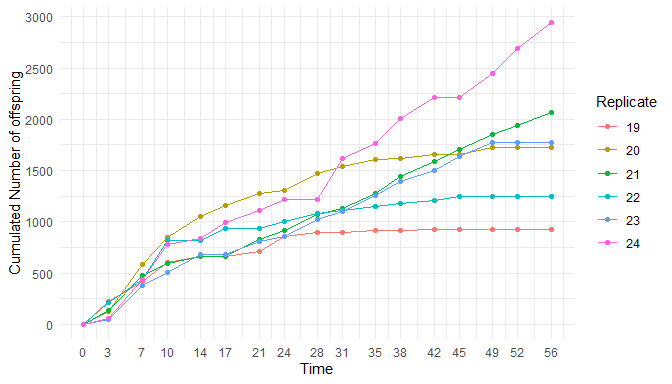# (5) represent the reproduction rate as a function of concentration
plotDoseResponse(dat, target.time = 28)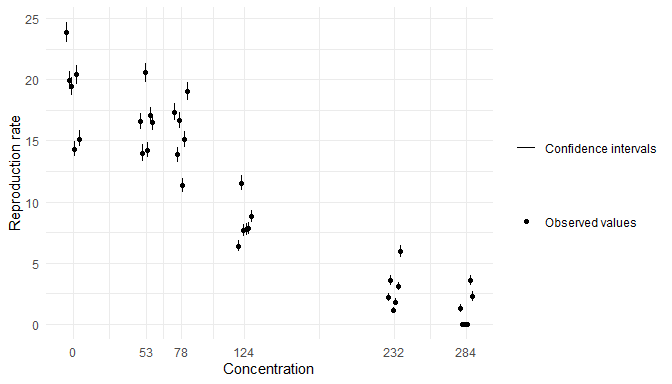# (6) check information on the experimental design
summary(dat)
##
## Number of replicates per time and concentration:
##      time
## conc  0 3 7 10 14 17 21 24 28 31 35 38 42 45 49 52 56
##   0   6 6 6  6  6  6  6  6  6  6  6  6  6  6  6  6  6
##   53  6 6 6  6  6  6  6  6  6  6  6  6  6  6  6  6  6
##   78  6 6 6  6  6  6  6  6  6  6  6  6  6  6  6  6  6
##   124 6 6 6  6  6  6  6  6  6  6  6  6  6  6  6  6  6
##   232 6 6 6  6  6  6  6  6  6  6  6  6  6  6  6  6  6
##   284 6 6 6  6  6  6  6  6  6  6  6  6  6  6  6  6  6
##
## Number of survivors (sum of replicates) per time and concentration:
##      0  3  7 10 14 17 21 24 28 31 35 38 42 45 49 52 56
## 0   30 30 30 30 29 29 29 29 29 28 28 28 28 28 28 28 28
## 53  30 30 29 29 29 29 29 29 29 29 28 28 28 28 28 28 28
## 78  30 30 30 30 30 30 29 29 29 29 29 29 29 29 29 27 27
## 124 30 30 30 30 30 29 28 28 27 26 25 23 21 18 11 11  9
## 232 30 30 30 22 18 18 17 14 13 12  8  4  3  1  0  0  0
## 284 30 30 15  7  4  4  4  2  2  1  1  1  1  1  1  0  0
##
## Number of offspring (sum of replicates) per time and concentration:
##     0    3    7   10   14   17   21   24   28   31   35   38   42   45   49
## 0   0 1659 1587 2082 1580 2400 2069 2316 1822 2860 2154 3200 1603 2490 1609
## 53  0 1221 1567 1710 1773 1859 1602 1995 1800 2101 1494 2126  935 1629 2108
## 78  0 1066 2023 1752 1629 1715 1719 1278 1717 1451 1826 1610 1097 1727 2309
## 124 0  807 1917 1423  567  383  568  493  605  631  573  585  546  280  594
## 232 0  270 1153  252   30    0   37   28   46  119   19    9    0    0    0
## 284 0  146  275   18    1    0    0    0    0    0    0    0    0    0    0
##       52   56
## 0   2149 2881
## 53  1686 1628
## 78  1954 1760
## 124  328  380
## 232    0    0
## 284    0    0
# (7) fit a concentration-effect model at target-time
fit <- reproFitTT(dat, stoc.part = "bestfit",
target.time = 21,
ecx = c(10, 20, 30, 40, 50),
quiet = TRUE)
summary(fit)
## Summary:
##
## The  loglogistic  model with a Gamma Poisson stochastic part was used !
##
## Priors on parameters (quantiles):
##
##             50%      2.5%     97.5%
## b     1.000e+00 1.259e-02 7.943e+01
## d     1.830e+01 1.554e+01 2.107e+01
## e     1.488e+02 7.902e+01 2.804e+02
## omega 1.000e+00 1.585e-04 6.310e+03
##
## Posteriors of the parameters (quantiles):
##
##             50%      2.5%     97.5%
## b     3.859e+00 2.835e+00 5.748e+00
## d     1.776e+01 1.547e+01 2.016e+01
## e     1.372e+02 1.144e+02 1.733e+02
## omega 1.442e+00 8.277e-01 2.778e+00
##
## Posteriors of the ECx (quantiles):
##
##            50%      2.5%     97.5%
## EC10 7.756e+01 5.475e+01 1.165e+02
## EC20 9.564e+01 7.223e+01 1.349e+02
## EC30 1.099e+02 8.679e+01 1.485e+02
## EC40 1.233e+02 1.006e+02 1.609e+02
## EC50 1.372e+02 1.144e+02 1.733e+02
plot(fit, log.scale = TRUE, adddata = TRUE,
cicol = "orange",
addlegend = TRUE)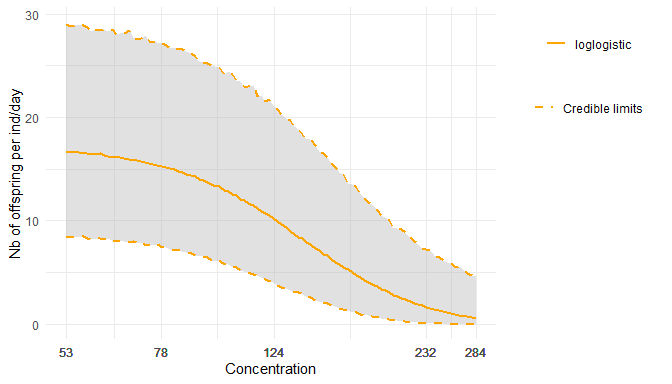ppc(fit)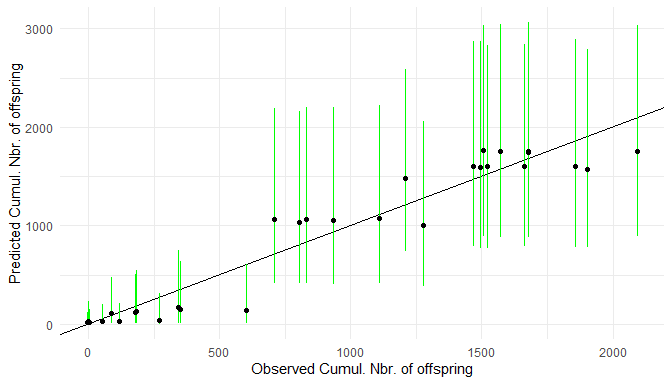As in the survival analysis, we assume that the reproduction rate per individual-day is a log-logistic function of the concentration. More details and parameter signification can be found in the modelling vignette.

## Model comparison

For reproduction analyses, we compare one model which neglects the inter-individual variability (named “Poisson”) and another one which takes it into account (named “gamma Poisson”). You can choose either one or the other with the option stoc.part. Setting this option to "bestfit", you let reproFitTT() decides which models fits the data best. The corresponding choice can be seen by calling the summary function:

summary(fit)
## Summary:
##
## The  loglogistic  model with a Gamma Poisson stochastic part was used !
##
## Priors on parameters (quantiles):
##
##             50%      2.5%     97.5%
## b     1.000e+00 1.259e-02 7.943e+01
## d     1.830e+01 1.554e+01 2.107e+01
## e     1.488e+02 7.902e+01 2.804e+02
## omega 1.000e+00 1.585e-04 6.310e+03
##
## Posteriors of the parameters (quantiles):
##
##             50%      2.5%     97.5%
## b     3.859e+00 2.835e+00 5.748e+00
## d     1.776e+01 1.547e+01 2.016e+01
## e     1.372e+02 1.144e+02 1.733e+02
## omega 1.442e+00 8.277e-01 2.778e+00
##
## Posteriors of the ECx (quantiles):
##
##            50%      2.5%     97.5%
## EC10 7.756e+01 5.475e+01 1.165e+02
## EC20 9.564e+01 7.223e+01 1.349e+02
## EC30 1.099e+02 8.679e+01 1.485e+02
## EC40 1.233e+02 1.006e+02 1.609e+02
## EC50 1.372e+02 1.144e+02 1.733e+02

When the gamma Poisson model is selected, the summary shows an additional parameter called omega, which quantifies the inter-individual variability (the higher omega the higher the variability).

## Reproduction data and survival functions

In morse, reproduction data sets are a special case of survival data sets: a reproduction data set includes the same information as in a survival data set plus the information on reproduction outputs. For that reason, the S3 class reproData inherits from the class survData, which means that any operation on a survData object is legal on a reproData object. In particular, in order to use the plot function related to the survival analysis on a reproData object, we can use survData as a conversion function first:

dat <- reproData(cadmium2)
plot(survData(dat))1. In Bayesian inference, the parameters of a model are estimated from the data starting from a so-called prior, which is a probability distribution representing an initial guess on the true parameters, before seing the data. The posterior distribution represents the uncertainty on the parameters after seeing the data and combining them with the prior. To obtain a point estimate of the parameters, it is typical to compute the mean or median of the posterior. We can quantify the uncertainty by reporting the standard deviation or an inter-quantile distance from this posterior distribution.↩︎